# Properties & Applications of Triangles in Geometry Chapter Exam

Exam Instructions:

Choose your answers to the questions and click 'Next' to see the next set of questions. You can skip questions if you would like and come back to them later with the yellow "Go To First Skipped Question" button. When you have completed the practice exam, a green submit button will appear. Click it to see your results. Good luck!

### Page 1

#### Question 1 1. In triangle GBN, Angle G is seven more than three times a number, Angle B is five more than four times the number, and Angle N is twelve less than five times the number. Solve for the measure of Angle G.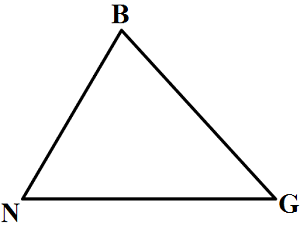#### Question 2 2. In the figure below, Angle 13 = 10y and Angle 11 = 3y + 11. Determine the measure of Angle 16.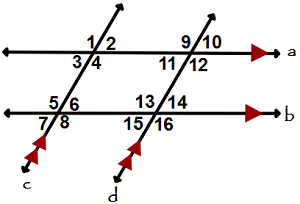#### Question 3 3. In the figure below, Angle 7 = 15x and Angle 10 = 9x + 30. Determine the measure of Angle 10.#### Question 4 4. Given the triangle ABC, solve for the value of y.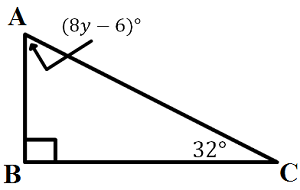#### Question 5 5. Given the triangle PQE, calculate the measure of Angle Q.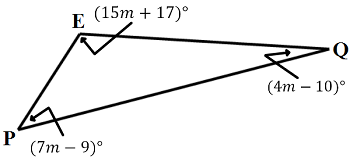### Page 2

#### Question 6 6. Given triangles DAR and KMR. What is the value of y?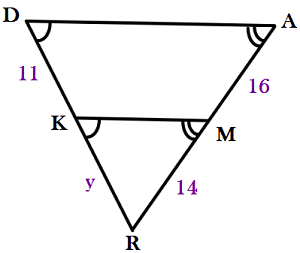#### Question 7 7. Study the given diagram. Find the value of x, the distance between T and E.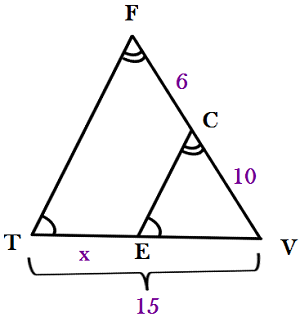#### Question 8 8. Triangle HYV and triangle AYB are similar by the AA similarity theorem. What is the value of x?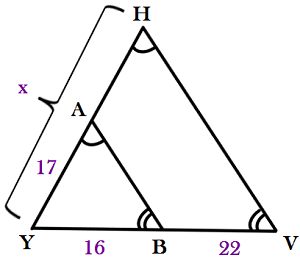### Page 3

#### Question 11 11. Conclude whether triangle BEN is similar to triangle MAT.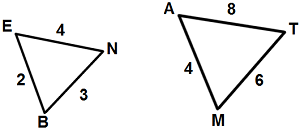#### Question 12 12. Determine if triangle VEZ is similar to triangle MAG.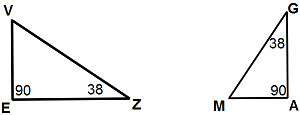#### Question 13 13. Josh claims that these triangles are similar by SSS. Do you agree or disagree with Josh?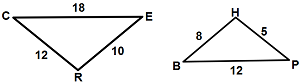#### Question 14 14. Mary claims that the triangles below are similar by AA. Do you agree or disagree with Mary?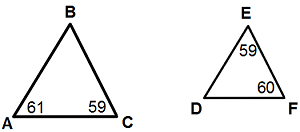#### Question 15 15. Is triangle ABC similar to triangle DEF?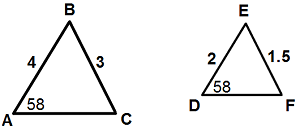### Page 4

#### Question 16 16. In the pictured triangle, what is side A, relative to angle x?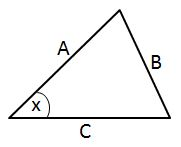#### Question 18 18. Identify x and y in the pictured triangle.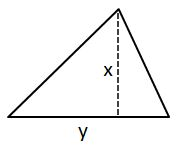#### Question 19 19. In the pictured triangle, what is side B, relative to angle x?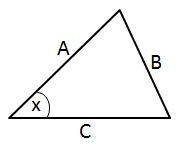### Page 5

#### Properties & Applications of Triangles in Geometry Chapter Exam Instructions

Choose your answers to the questions and click 'Next' to see the next set of questions. You can skip questions if you would like and come back to them later with the yellow "Go To First Skipped Question" button. When you have completed the practice exam, a green submit button will appear. Click it to see your results. Good luck!

Support